### Home > PC > Chapter 6 > Lesson 6.1.2 > Problem6-29

6-29.
1.. Evaluate each expression for θ (in radians) and leave your answers in fraction form when appropriate. Remember the restricted ranges for the functions. Homework Help ✎

1. θ = sin−1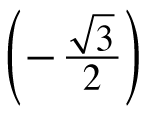2. θ = arcsin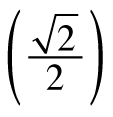3. θ = arccos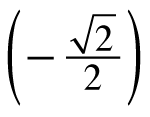4. θ = cos−1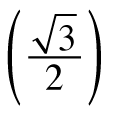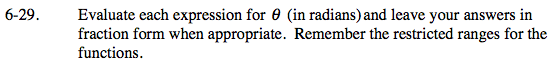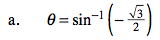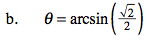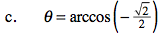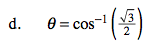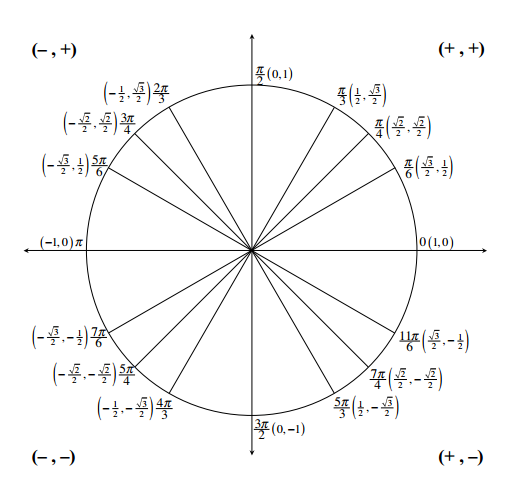$\text{The domain of inverse sine is }\left[-\frac{\pi}{2},\frac{\pi}{2}\right]. \text{The domain of inverse cosine is }[0,\pi].$